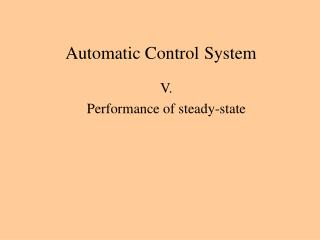DownloadDownload PresentationAutomatic Control System

# Automatic Control System

Download Presentation## Automatic Control System

- - - - - - - - - - - - - - - - - - - - - - - - - - - E N D - - - - - - - - - - - - - - - - - - - - - - - - - - -
##### Presentation Transcript

1. Automatic ControlSystem V. Performance of steady-state

2. Terms of feedback control controller plant controlled variable compensator or control task transmitter manipulated variable error signal actuator comparing element or error detector disturbance variable reference signal feedback signal reference input element block model of the plant action signal

3. Working points in steady-state W In steady-state the value of the action signal U, manipulated UM, and controlled Y variables, and the feedback signal YM are constant. UM U YM Y Y In steady-state the output of a loop’s element is the input of the next block. The steady-state characteristics of the elements must be fitted each other. The disturbance w variable’s deviation of the average value changes the plant’s steady-state characteristic curve. It’s important: A new steady-state can be achieved by the system. W0 YM UM U

4. Choosing steady-state characteristics of controller The steady-state characteristic of the plant, transmitter and the actuator is given by the technologies. We can choose only the steady-statecharacteristic of the controller. If higher action signal belongs to higher feedback signal then the characteristic is direct. If lower action signal belongs to higher feedback signal then the characteristic is reverse. The dynamic behavior of the controller eliminates the steady-state error.. Y W0 UM YM U In this case there isn’t a new steady-state value of system.

5. Choosing steady-state characteristics of controller A bad choice of controller steady-state characteristics also causes an unstable control loop. Y W0 Sometimes the technologies requirements need a reverse characteristics of the actuator. Sometimes the steady-state characteristics of technologies is concave and not convex. YM UM U In this case there is a new steady-state value of system.

6. GA(s) Transfer functions of feedback loop GW(s) GR(s) GP(s) GC(s) GT(s)

7. GA(s) System type of feedback loop GW(s) GR(s) GP(s) GC(s) GT(s) Opened-loop transfer function, Bode form. System type is the number of the pure integral lag in the loop. The possible values are 0, 1, 2.K0 is the loop gain.

8. Example A ship wants to turn to the port. The continuous movement of the steering wheel turns the ship. The surface of the ship against the river backwash also continuously increase.How much is the deflection error if r(t)=0.2*t*1(t) is the changes of the reference signal and w(t)=0.1*t*1(t) is the changes of the disturbance variable. The domains are: Y = ± 80 [°], R = 4 – 20 [mA]. v

9. The steady-state error of closed loop control

10. The steady-state error of closed loop control The size of the error might be appropriate, but we do not know anything about the quality characteristics.

11. Setpoint tracking The setpoint tracking means that the controlled variable (y(s)) is able to follow the prescribed value by the changes of the reference signal (r(s)) after the transient has died. We assume that the disturbance variable (W0(s)) equals the designed value (w(s)=0). We can examine this with the error transfer function: • The setpoint tracking depends on: • Order of the feedback system. • The loop‘s gain (K0). • The form of the reference signal

12. Setpoint tracking Order of the feedback system Standard signal 0 1 2 0 0 t 0 t t

13. Disturbance suppression The disturbance suppression means that the controlled variable (y(s)) is able to hold the prescribed value by the changes of the disturbance variable (w(s)) after the transient has died. We assume that the reference signal (R0(s)) equals the working point value (r(s)=0). We can examine this with the disturbance-error transfer function: • The disturbance suppression depends on: • Order of the feedback system. • The loop‘s gain (K0). • The form of the reference signal. • The attack point of the disturbance variable.

14. Disturbance suppression Order of the feedback system 0 1 2 Entering point of disturbance Standard signal 0 0 1 0 1 2 0 0 0 t 0 t t